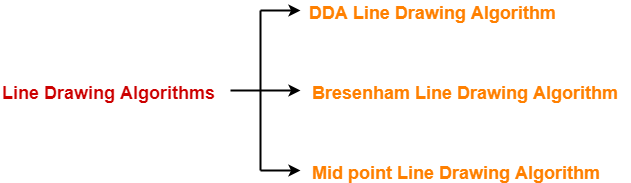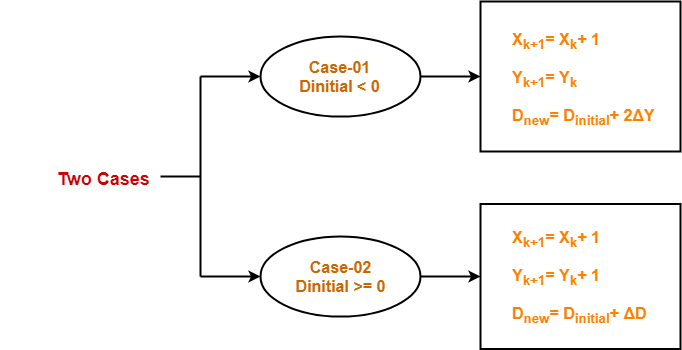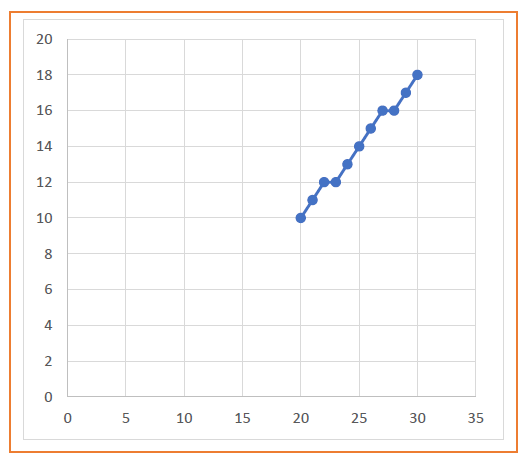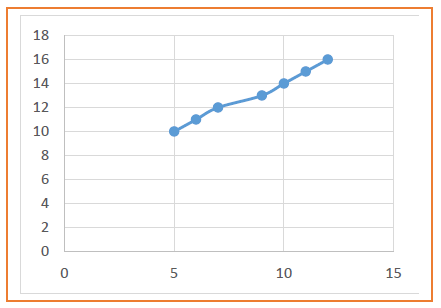## Line Drawing Algorithms-

In computer graphics, popular algorithms used to generate lines are-1. Digital Differential Analyzer (DDA) Line Drawing Algorithm
2. Bresenham Line Drawing Algorithm
3. Mid Point Line Drawing Algorithm

## Mid Point Line Drawing Algorithm-

 Given the starting and ending coordinates of a line,Mid Point Line Drawing Algorithm attempts to generate the points between the starting and ending coordinates.

Also Read- DDA Line Drawing Algorithm

### Procedure-

Given-

• Starting coordinates = (X0, Y0)
• Ending coordinates = (Xn, Yn)

The points generation using Mid Point Line Drawing Algorithm involves the following steps-

### Step-01:

Calculate ΔX and ΔY from the given input.

These parameters are calculated as-

• ΔX = Xn – X0
• ΔY =Yn – Y0

### Step-02:

Calculate the value of initial decision parameter and ΔD.

These parameters are calculated as-

• Dinitial = 2ΔY – ΔX
• ΔD = 2(ΔY – ΔX)

### Step-03:

The decision whether to increment X or Y coordinate depends upon the flowing values of Dinitial.### Step-04:

Keep repeating Step-03 until the end point is reached.

For each Dnew value, follow the above cases to find the next coordinates.

## Problem-01:

Calculate the points between the starting coordinates (20, 10) and ending coordinates (30, 18).

## Solution-

Given-

• Starting coordinates = (X0, Y0) = (20, 10)
• Ending coordinates = (Xn, Yn) = (30, 18)

### Step-01:

Calculate ΔX and ΔY from the given input.

• ΔX = Xn – X0 = 30 – 20 = 10
• ΔY =Yn – Y0 = 18 – 10 = 8

### Step-02:

Calculate Dinitial and ΔD as-

• Dinitial = 2ΔY – ΔX = 2 x 8 – 10 = 6
• ΔD = 2(ΔY – ΔX) = 2 x (8 – 10) = -4

### Step-03:

As Dinitial >= 0, so case-02 is satisfied.

Thus,

• Xk+1 = Xk + 1 = 20 + 1 = 21
• Yk+1 = Yk + 1 = 10 + 1 = 11
• Dnew = Dinitial + ΔD = 6 + (-4) = 2

Similarly, Step-03 is executed until the end point is reached.

 Dinitial Dnew Xk+1 Yk+1 20 10 6 2 21 11 2 -2 22 12 -2 14 23 12 14 10 24 13 10 6 25 14 6 2 26 15 2 -2 27 16 -2 14 28 16 14 10 29 17 10 30 18## Problem-02:

Calculate the points between the starting coordinates (5, 9) and ending coordinates (12, 16).

## Solution-

Given-

• Starting coordinates = (X0, Y0) = (5, 9)
• Ending coordinates = (Xn, Yn) = (12, 16)

### Step-01:

Calculate ΔX and ΔY from the given input.

• ΔX = Xn – X0 = 12 – 5 = 7
• ΔY =Yn – Y0 = 16 – 9 = 7

### Step-02:

Calculate Dinitial and ΔD as-

• Dinitial = 2ΔY – ΔX = 2 x 7 – 7 = 7
• ΔD = 2(ΔY – ΔX) = 2 x (7 – 7) = 0

### Step-03:

As Dinitial >= 0, so case-02 is satisfied.

Thus,

• Xk+1 = Xk + 1 = 5 + 1 = 6
• Yk+1 = Yk + 1 = 9 + 1 = 10
• Dnew = Dinitial + ΔD = 7 + 0 = 7

Similarly, Step-03 is executed until the end point is reached.

 Dinitial Dnew Xk+1 Yk+1 5 9 7 7 6 10 7 7 7 11 7 7 8 12 7 7 9 13 7 7 10 14 7 7 11 15 7 12 16## Advantages of Mid Point Line Drawing Algorithm-

The advantages of Mid Point Line Drawing Algorithm are-

• Accuracy of finding points is a key feature of this algorithm.
• It is simple to implement.
• It uses basic arithmetic operations.
• It takes less time for computation.
• The resulted line is smooth as compared to other line drawing algorithms.

Also Read- Bresenham Line Drawing Algorithm

## Disadvantages of Mid Point Line Drawing Algorithm-

The disadvantages of Mid Point Line Drawing Algorithm are-

• This algorithm may not be an ideal choice for complex graphics and images.
• In terms of accuracy of finding points, improvement is still needed.
• There is no any remarkable improvement made by this algorithm.

To gain better understanding about Mid Point Line Drawing Algorithm,

Watch this Video Lecture

Next Article- Mid Point Circle Drawing Algorithm

Get more notes and other study material of Computer Graphics.

Watch video lectures by visiting our YouTube channel LearnVidFun.